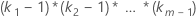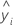# Methods and formulas for analysis of variance in Fit General Linear Model

Select the method or formula of your choice.

## Sum of squares (SS)

In matrix terms, these are the formulas for the different sums of squares:

Minitab breaks down the SS Regression or SS Treatments component into the amount of variation explained by each term using both the sequential sum of squares and adjusted sum of squares.

### Notation

TermDescription
bvector of coefficients
Xdesign matrix
Yvector of response values
nnumber of observations
Jn by n matrix of 1s

## Sequential sum of squares

Minitab breaks down the SS Regression or Treatments component of variance into sequential sums of squares for each factor. The sequential sums of squares depend on the order the factors or predictors are entered into the model. The sequential sum of squares is the unique portion of SS Regression explained by a factor, given any previously entered factors.

For example, if you have a model with three factors or predictors, X1, X2, and X3, the sequential sum of squares for X2 shows how much of the remaining variation X2 explains, given that X1 is already in the model. To obtain a different sequence of factors, repeat the analysis and enter the factors in a different order.

## Adjusted sum of squares

The adjusted sums of squares does not depend on the order that the terms enter the model. The adjusted sum of squares is the amount of variation explained by a term, given all other terms in the model, regardless of the order that the terms enter the model.

For example, if you have a model with three factors, X1, X2, and X3, the adjusted sum of squares for X2 shows how much of the remaining variation the term for X2 explains, given that the terms for X1 and X3 are also in the model.

The calculations for the adjusted sums of squares for three factors are:

• SSR(X3 | X1, X2) = SSE (X1, X2) - SSE (X1, X2, X3) or
• SSR(X3 | X1, X2) = SSR (X1, X2, X3) - SSR (X1, X2)

where SSR(X3 | X1, X2) is the adjusted sum of squares for X3, given that X1 and X2 are in the model.

• SSR(X2, X3 | X1) = SSE (X1) - SSE (X1, X2, X3) or
• SSR(X2, X3 | X1) = SSR (X1, X2, X3) - SSR (X1)

where SSR(X2, X3 | X1) is the adjusted sum of squares for X2 and X3, given that X1 is in the model.

You can extend these formulas if you have more than 3 factors in your model1.

1. J. Neter, W. Wasserman and M.H. Kutner (1985). Applied Linear Statistical Models, Second Edition. Irwin, Inc.

## Degrees of freedom (DF)

The degrees of freedom for each component of the model are:

Sources of variation DF
Factor ki – 1
Covariates and interactions between covariates 1
Interactions that involve factorsRegression p
Error n p – 1
Total n – 1

If your data meet certain criteria and the model includes at least one continuous predictor or more than one categorical predictor, then Minitab uses some degrees of freedom for the lack-of-fit test. The criteria are as follows:
• The data contain multiple observations with the same predictor values.
• The data contain the correct points to estimate additional terms that are not in the model.

### Notation

TermDescription
kinumber of levels in the ith factor
mnumber of factors
n number of observations
p number of coefficients in the model, not counting the constant

## Adj MS – Regression

The formula for the Mean Square (MS) of the regression is:

### Notation

TermDescriptionmean responseith fitted response
pnumber of terms in the model

## Adj MS – Error

The Mean Square of the error (also abbreviated as MS Error or MSE, and denoted as s2) is the variance around the fitted regression line. The formula is:

### Notation

TermDescription
yiith observed response valueith fitted response
nnumber of observations
pnumber of coefficients in the model, not counting the constant

## F

If all the factors in the model are fixed, then the calculation of the F-statistic depends on what the hypothesis test is about, as follows:

F(Term)
F(Lack-of-fit)

If there are random factors in the model, F is constructed using the expected mean square information for each term. For more information, see Neter et al.1.

### Notation

TermDescription
Adj MS TermA measure of the amount of variation that a term explains after accounting for the other terms in the model.
MS ErrorA measure of the variation that the model does not explain.
MS Lack-of-fitA measure of variation in the response that could be modeled by adding more terms to the model.
MS Pure errorA measure of the variation in replicated response data.
1. J. Neter, W. Wasserman and M.H. Kutner (1985). Applied Linear Statistical Models, Second Edition. Irwin, Inc.

## P-value – Analysis of variance table

The p-value is a probability that is calculated from an F-distribution with the degrees of freedom (DF) as follows:

Numerator DF
sum of the degrees of freedom for the term or the terms in the test
Denominator DF
degrees of freedom for error

1 − P(Ffj)

### Notation

TermDescription
P(Ff)cumulative distribution function for the F-distribution
ff-statistic for the test

## Pure error lack-of-fit test

To calculate the pure error lack-of-fit test, Minitab calculates:
1. The sum of squared deviations of the response from the mean within each set of replicates and adds them together to create the pure error sum of squares (SS PE).
2. The pure error mean square

where n = number of observations and m = number of distinct x-level combinations

3. The lack-of-fit sum of squares
4. The lack-of-fit mean square
5. The test statistics

Large F-values and small p-values suggest that the model is inadequate.

## P-value – Lack-of-fit test

This p-value is for the test of the null hypothesis that the coefficients are 0 for any terms that are possible to estimate from these data that are not in the model. The p-value is the probability from an F distribution with degrees of freedom (DF) as follows:
Numerator DF
degrees of freedom for lack-of-fit
Denominator DF
degrees of freedom for pure error

1 − P(Ffj)

### Notation

TermDescription
P(Ffj)cumulative distribution function for the F-distribution
fjf-statistic for the test
By using this site you agree to the use of cookies for analytics and personalized content.  Read our policy